# HSSlive: Plus One & Plus Two Notes & Solutions for Kerala State Board

## AP Board Class 7 Maths Chapter 5 Triangle and Its Properties InText Questions Textbook Solutions PDF: Download Andhra Pradesh Board STD 7th Maths Chapter 5 Triangle and Its Properties InText Questions Book AnswersAP Board Class 7 Maths Chapter 5 Triangle and Its Properties InText Questions Textbook Solutions PDF: Download Andhra Pradesh Board STD 7th Maths Chapter 5 Triangle and Its Properties InText Questions Book Answers

## Andhra Pradesh State Board Class 7th Maths Chapter 5 Triangle and Its Properties InText Questions Books Solutions

 Board AP Board Materials Textbook Solutions/Guide Format DOC/PDF Class 7th Subject Maths Chapters Maths Chapter 5 Triangle and Its Properties InText Questions Provider Hsslive

## How to download Andhra Pradesh Board Class 7th Maths Chapter 5 Triangle and Its Properties InText Questions Textbook Solutions Answers PDF Online?

2. Click on the Andhra Pradesh Board Class 7th Maths Chapter 5 Triangle and Its Properties InText Questions Answers.
3. Look for your Andhra Pradesh Board STD 7th Maths Chapter 5 Triangle and Its Properties InText Questions Textbooks PDF.
4. Now download or read the Andhra Pradesh Board Class 7th Maths Chapter 5 Triangle and Its Properties InText Questions Textbook Solutions for PDF Free.

## AP Board Class 7th Maths Chapter 5 Triangle and Its Properties InText Questions Textbooks Solutions with Answer PDF Download

Find below the list of all AP Board Class 7th Maths Chapter 5 Triangle and Its Properties InText Questions Textbook Solutions for PDF’s for you to download and prepare for the upcoming exams:

Try This

Question 1.
The lengths of two sides of a triangle are 6 cm and 9 cpi. Write all the possible lengths of the third side. (Page No. 92)
Solution:
The possible length of the third side must greater than (9— 6) and less than (9 + 6).
∴ The third side may be 4 cm, 5 cm, 6 cm, 7 cm, 8 cm, 9 cm, 10 cm, 11 cm, 12 cm, 13 cm, 14 cm.

Try This

Question 1.
Uma felt that a triangle can be formed with three collinear points. Do you agree ? Why? Draw diagrams to justify your answer. (Page No. 88)
Solution:
Uma ¡s not correct.
A triangle cant be formed with three collinear points.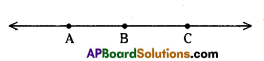Do This

Question 1.
Classify the following triangles according to their (i) sides and (ii) angles. (Page No. 89)
Solution: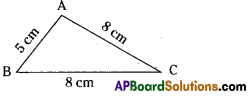Two sides AC and BC are equal.
∴ ∆ABC is an isosceles triangle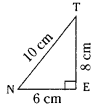As ∠E = 90°;
∆NET is a right angled triangle.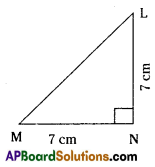In ∆MNL,
MN = NL and ∠N = 90°
∆ MNL is right angled isosceless triangle.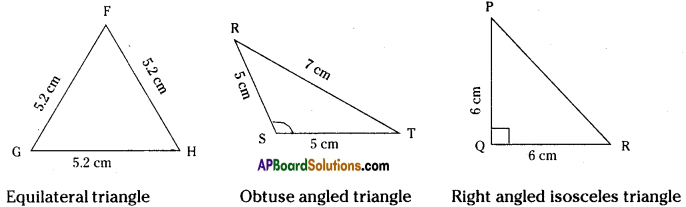Question 2.
Write the six elements (i.e., the 3 sides and 3 angles) of ∆ABC.
Solution:
The three sides AB⎯⎯⎯⎯⎯⎯⎯⎯,BC⎯⎯⎯⎯⎯⎯⎯⎯,CA⎯⎯⎯⎯⎯⎯⎯⎯ and the three angles ∠A, ∠B and ∠C are the six elements of ∆ABC.

Question 3.
Write the side opposite to vertex Q in ∆PQR.
Solution:
The side opposite to the vertex Q is  PR  .

Question 4.
Write the angle opposite to side  LM  in ∆LMN.
Solution:
Angle opposite to side  LM  is ∠N.

Question 5.
Write the vertex opposite to side  RT  in ∆RST.
Solution:
Vertex opposite to  RT  is S.

Try This

Question 1.
Rashmi claims that no triangle can have more than one right angle. Do you agree with her. Why ?
Solution:
Yes, Rashmi is correct. A triangle can’t have more than one right angle. As the sum of two right angles is 180° there will be no scope for 3rd angle.

Question 2.
Kamal claims that no triangle can have more than two acute angles. Do you agree with him. Why?
Solution:
No. Kamal is not right. A triangle can have all the three acute angles.
(60°, 60°, 60°), (30°, 70°, 80°), (40°, 60°, 80°) etc ………….

Question 3 i)
Draw altitudes from P to  QR  for the following triangles. Also, draw altitudes from the other vertices.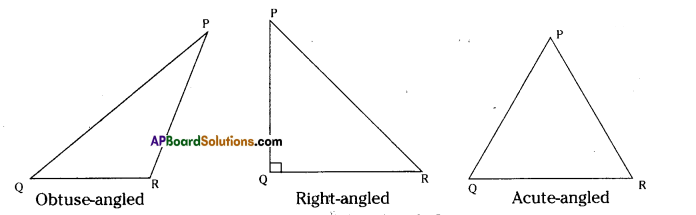ii) Will an altitude always lie in the interior of a triangle ?
iii) Can you think of a triangle in which the two altitudes of a triangle are two of its sides ?
Solution:
i)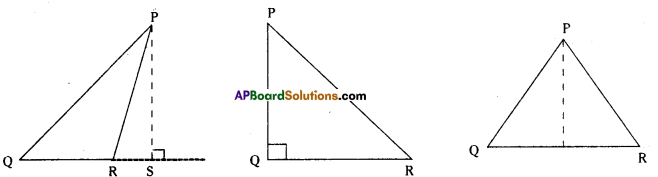ii) An altitude need not necessarily be in the Interior of a triangle.
iii) Yes. In a right triangle the two sides containing the right angle are the two altitudes of it.

Do This

Question 1.
Draw ∆ABC and fofm an exterior ∠ACD. Now take a protractor and measure ∠ACD, ∠A and ∠B. Find the sum ∠A + ∠B and compare it with the measure ∠ACD. Do you observe that ZACD is equal (or nearly equal) to ∠A + ∠B ? (Page No. 102)
Solution:
For instance in the adjacent figure
∠A = 68° ; ∠B = 57°
∠A + ∠B = 68° + 57° = 125 ∠ACD = 125°
∴ ∠ACD = ∠A + ∠B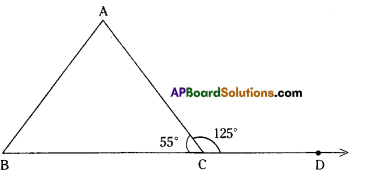Question 2.
Copy each of the following triangles. In each case verify that an exterior angle of a triangle^ is equal to the sum of the two interior opposite angles. (Page No. 103)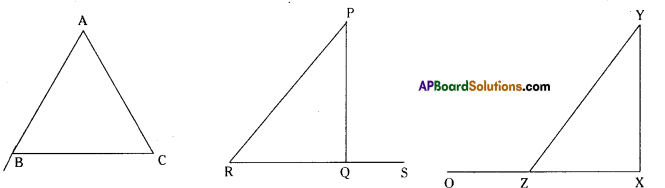Solution:
From the figures
exterior ∠B = ∠A + ∠C
∠130° = 65° + 65°
∠PQS = ∠P + ∠R also ∠YZO = ∠X
90° = 40° + 50° 120° = 90° + 30°

## Andhra Pradesh Board Class 7th Maths Chapter 5 Triangle and Its Properties InText Questions Textbooks for Exam Preparations

Andhra Pradesh Board Class 7th Maths Chapter 5 Triangle and Its Properties InText Questions Textbook Solutions can be of great help in your Andhra Pradesh Board Class 7th Maths Chapter 5 Triangle and Its Properties InText Questions exam preparation. The AP Board STD 7th Maths Chapter 5 Triangle and Its Properties InText Questions Textbooks study material, used with the English medium textbooks, can help you complete the entire Class 7th Maths Chapter 5 Triangle and Its Properties InText Questions Books State Board syllabus with maximum efficiency.

## FAQs Regarding Andhra Pradesh Board Class 7th Maths Chapter 5 Triangle and Its Properties InText Questions Textbook Solutions

#### Can we get a Andhra Pradesh State Board Book PDF for all Classes?

Yes you can get Andhra Pradesh Board Text Book PDF for all classes using the links provided in the above article.

## Important Terms

Andhra Pradesh Board Class 7th Maths Chapter 5 Triangle and Its Properties InText Questions, AP Board Class 7th Maths Chapter 5 Triangle and Its Properties InText Questions Textbooks, Andhra Pradesh State Board Class 7th Maths Chapter 5 Triangle and Its Properties InText Questions, Andhra Pradesh State Board Class 7th Maths Chapter 5 Triangle and Its Properties InText Questions Textbook solutions, AP Board Class 7th Maths Chapter 5 Triangle and Its Properties InText Questions Textbooks Solutions, Andhra Pradesh Board STD 7th Maths Chapter 5 Triangle and Its Properties InText Questions, AP Board STD 7th Maths Chapter 5 Triangle and Its Properties InText Questions Textbooks, Andhra Pradesh State Board STD 7th Maths Chapter 5 Triangle and Its Properties InText Questions, Andhra Pradesh State Board STD 7th Maths Chapter 5 Triangle and Its Properties InText Questions Textbook solutions, AP Board STD 7th Maths Chapter 5 Triangle and Its Properties InText Questions Textbooks Solutions,
Share: